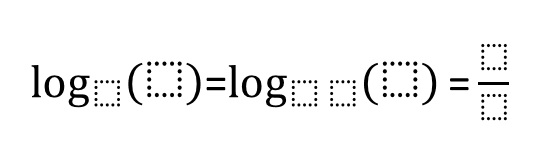# Logarithms 1-B

Directions: Using the digits 0 to 9 at most one time each, place a digit in each box to make the statement true.### Hint

Were you able to build off the first equation to solve the second? If you’ve found one solution, can you find another? Does the first equation have more solutions than the second?